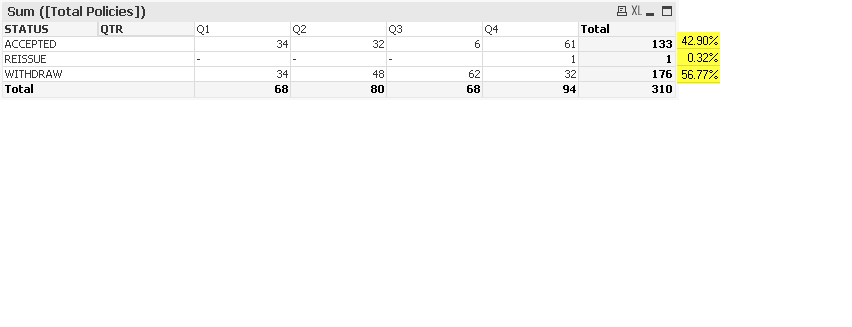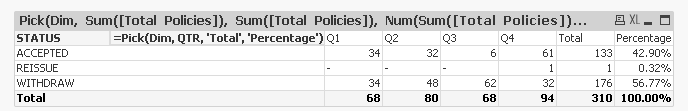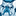# QlikView App Dev

Discussion Board for collaboration related to QlikView App Development.

Announcements
Action-Packed Learning Awaits! QlikWorld 2023. April 17 - 20 in Las Vegas: REGISTER NOW
cancel
Showing results for
Did you mean:Creator II

## help with Pivot

Hi,

I am using a pivot in one of my apps. Wanted to know if the following is possible.

I want a new column for  the percentages to be displayed in pivot like in following image.133/310*100= 42.9%

1/310*100= 0.32%

176/310*100=56.77%

Please advise if this is possible in pivot.(Attached is the qvw and excel with test data)

1 Solution

Accepted SolutionsMVP

May be like this:

QTR,

STATUS,

[Total Policies]

FROM

(ooxml, embedded labels, table is Sheet1);

Dim

1

2

3

];

Pivot Table

Dimension

1) STATUS

2) =Pick(Dim, QTR, 'Total', 'Percentage')

Expressions

Pick(Dim, Sum([Total Policies]), Sum([Total Policies]), Num(Sum([Total Policies])/Sum(TOTAL [Total Policies]), '##.##%'))2 RepliesMaster II

I don't think it is possible with Pivot chart, But it is possible with Straight Table. See the attached file:

Hope this helps...MVP

May be like this:

QTR,

STATUS,

[Total Policies]

FROM

(ooxml, embedded labels, table is Sheet1);

Dim

1

2

3

];

Pivot Table

Dimension

1) STATUS

2) =Pick(Dim, QTR, 'Total', 'Percentage')

Expressions

Pick(Dim, Sum([Total Policies]), Sum([Total Policies]), Num(Sum([Total Policies])/Sum(TOTAL [Total Policies]), '##.##%'))Community Browser